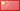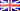中文版ENGLISH
What is the efficiency of Inverter? Continuous Power? Peak Power?
What is the efficiency of Inverter?

A part of power will be consumed while inverter is working. Therefore, its input power should larger than its output power.
Efficiency is the ratio of input power to output power.
For example, if the input power is 100W, output power is 90W, then efficiency is 90%.

What is Continuous output power? What is peak power?

Some appliances or tools with a motor, such as refrigerator, washing machine, drill ect. draw a very high surge of current during
start up, but
once started, only smaller current is required for its operation. Therefore, Inverter has continuous output power and
peak power. Continuous output power means the rated power; in generally, peak power is twice than rated power. Must stress
that some appliances like air conditioner, refrigerator ect. Their starting current is as 3-7 times as the normal working current.
Thus, only the inverters which can meet the appliance’s starting peak power can work normally.